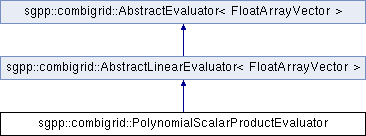SG++-Doxygen-Documentation
sgpp::combigrid::PolynomialScalarProductEvaluator Class Reference

This evaluator calculates the scalar products $$\int b_i b_j$$. More...

#include <PolynomialScalarProductEvaluator.hpp>

Inheritance diagram for sgpp::combigrid::PolynomialScalarProductEvaluator:## Public Member Functions

std::shared_ptr< AbstractLinearEvaluator< FloatArrayVector > > cloneLinear () override
Clones this object and returns it as a shared pointer to AbstractLinearEvaluator<V>. More...

std::vector< double > getBasisCoefficients () override

std::vector< FloatArrayVectorgetBasisValues () override
This method is specific to AbstractLinearEvaluator and has to be implemented by all subclasses. More...

void initializeBounds (std::shared_ptr< sgpp::combigrid::OrthogonalPolynomialBasis1D > orthogBasis)

bool needsOrderedPoints () override

bool needsParameter () override

PolynomialScalarProductEvaluator ()

PolynomialScalarProductEvaluator (sgpp::combigrid::SingleFunction weight_function, bool normalizeWeights=true, size_t numAdditionalPoints=10)

PolynomialScalarProductEvaluator (std::shared_ptr< sgpp::combigrid::OrthogonalPolynomialBasis1D > orthogBasis)

PolynomialScalarProductEvaluator (PolynomialScalarProductEvaluator const &other)

void setBasisCoefficientsAtGridPoints (std::vector< double > &functionValues) override
set the basis coefficients for the linear combination. More...

void setGridPoints (std::vector< double > const &newXValues) override
Sets the grid points for evaluation. More...

void setParameter (FloatArrayVector const &param) override
Via this method, the parameter can be set. More...

virtual ~PolynomialScalarProductEvaluator ()Public Member Functions inherited from sgpp::combigrid::AbstractLinearEvaluator< FloatArrayVector >
virtual std::shared_ptr< AbstractEvaluator< FloatArrayVector > > clone ()
Clones the AbstractEvaluator object. More...

virtual FloatArrayVector eval ()
AbstractLinearEvaluator provides a standard implementation of this method based on getBasisCoefficients(). More...

virtual void getBounds (double &a, double &b)

virtual void getWeightFunction (sgpp::combigrid::SingleFunction &weight_function)

virtual bool hasCustomWeightFunction ()

virtual void setBounds (double a, double b)

virtual void setWeightFunction (sgpp::combigrid::SingleFunction weight_function)

virtual ~AbstractLinearEvaluator ()Public Member Functions inherited from sgpp::combigrid::AbstractEvaluator< FloatArrayVector >
virtual EvaluatorConfiguration getConfig ()
(Currently not used). More...

virtual void setConfig (EvaluatorConfiguration newEvalConfig)

void setLevel (size_t level)
This is used to set the level in case an evaluator needs it. More...

virtual ~AbstractEvaluator ()Protected Attributes inherited from sgpp::combigrid::AbstractEvaluator< FloatArrayVector >
EvaluatorConfiguration evalConfig

size_t level

## Detailed Description

This evaluator calculates the scalar products $$\int b_i b_j$$.

This is done via quadrature based on the given grid points. The quadrature weights are obtained by (numerically) integrating the Lagrange polynomials on the given grid points. In the constructor, a weight function may be passed whose values at the grid points are multiplied with the given function values.

## ◆ PolynomialScalarProductEvaluator() [1/4]

 sgpp::combigrid::PolynomialScalarProductEvaluator::PolynomialScalarProductEvaluator ( )

## ◆ PolynomialScalarProductEvaluator() [2/4]

 sgpp::combigrid::PolynomialScalarProductEvaluator::PolynomialScalarProductEvaluator ( sgpp::combigrid::SingleFunction weight_function, bool normalizeWeights = true, size_t numAdditionalPoints = 10 )
Parameters
 numAdditionalPoints Specifies how many Gauss-Legrendre points should be used in addition to the default when integrating the Lagrange polynomials for computing the quadrature weights. This number should be higher if the weight function is hard to integrate. weight_function optional weight function w that can be included in the quadrature weights. The Quadrature evaluator then approximates the integral of f*w instead of the integral of f. This provides more precision by calling w more often than f, which might be useful if w can be evaluated much faster than f. normalizeWeights If this is set to true, the weights are normalized so that they sum up to 1. This might be useful if the weight function is (or should be) a probability distribution on the domain.

## ◆ PolynomialScalarProductEvaluator() [3/4]

 sgpp::combigrid::PolynomialScalarProductEvaluator::PolynomialScalarProductEvaluator ( std::shared_ptr< sgpp::combigrid::OrthogonalPolynomialBasis1D > orthogBasis )

## ◆ PolynomialScalarProductEvaluator() [4/4]

 sgpp::combigrid::PolynomialScalarProductEvaluator::PolynomialScalarProductEvaluator ( PolynomialScalarProductEvaluator const & other )

## ◆ ~PolynomialScalarProductEvaluator()

 sgpp::combigrid::PolynomialScalarProductEvaluator::~PolynomialScalarProductEvaluator ( )
virtual

## ◆ cloneLinear()

 std::shared_ptr< AbstractLinearEvaluator< FloatArrayVector > > sgpp::combigrid::PolynomialScalarProductEvaluator::cloneLinear ( )
overridevirtual

Clones this object and returns it as a shared pointer to AbstractLinearEvaluator<V>.

Referenced by getBasisCoefficients().

## ◆ getBasisCoefficients()

 std::vector sgpp::combigrid::PolynomialScalarProductEvaluator::getBasisCoefficients ( )
inlineoverridevirtual

## ◆ getBasisValues()

 std::vector sgpp::combigrid::PolynomialScalarProductEvaluator::getBasisValues ( )
inlineoverridevirtual

This method is specific to AbstractLinearEvaluator and has to be implemented by all subclasses.

It should return the coefficients used for the linear combination of function values. Example: If you want to do a linear interpolation and have the grid points 0.0 and 0.5 and an evaluation point (the parameter) of 0.2, you would return the weights (0.6, 0.4), because the interpolated value is 0.6 * f(0) + 0.4 * f(0.5).

In the case of multi-evaluation, this returns several coefficients for each grid point. Continuing the example above, if you would have evaluation points 0.2 and 0.4, the basis coefficients returned should be ((0.6, 0.2), (0.4, 0.8)).

## ◆ initializeBounds()

 void sgpp::combigrid::PolynomialScalarProductEvaluator::initializeBounds ( std::shared_ptr< sgpp::combigrid::OrthogonalPolynomialBasis1D > orthogBasis )

## ◆ needsOrderedPoints()

 bool sgpp::combigrid::PolynomialScalarProductEvaluator::needsOrderedPoints ( )
overridevirtual
Returns
true iff the grid points have to be provided in ascending order (for example for linear interpolation).

Referenced by getBasisCoefficients().

## ◆ needsParameter()

 bool sgpp::combigrid::PolynomialScalarProductEvaluator::needsParameter ( )
overridevirtual
Returns
true iff this evaluation method needs a parameter (e.g. true for interpolation, false for quadrature).

Referenced by getBasisCoefficients().

## ◆ setBasisCoefficientsAtGridPoints()

 void sgpp::combigrid::PolynomialScalarProductEvaluator::setBasisCoefficientsAtGridPoints ( std::vector< double > & newBasisCoefficients )
overridevirtual

set the basis coefficients for the linear combination.

In case of linear or polynomial interpolation these basis coefficients are function evaluations

Parameters
 newBasisCoefficients the new coefficients

References python.statsfileInfo::i, and python.utils.statsfile2gnuplot::j.

Referenced by getBasisCoefficients().

## ◆ setGridPoints()

 void sgpp::combigrid::PolynomialScalarProductEvaluator::setGridPoints ( std::vector< double > const & xValues )
overridevirtual

Sets the grid points for evaluation.

The evaluation method should allow for arbitrary grid points to achieve maximal modularity.

References python.statsfileInfo::i, and python.utils.statsfile2gnuplot::j.

Referenced by getBasisCoefficients().

## ◆ setParameter()

 void sgpp::combigrid::PolynomialScalarProductEvaluator::setParameter ( FloatArrayVector const & param )
overridevirtual

Via this method, the parameter can be set.

Referenced by getBasisCoefficients().

The documentation for this class was generated from the following files: